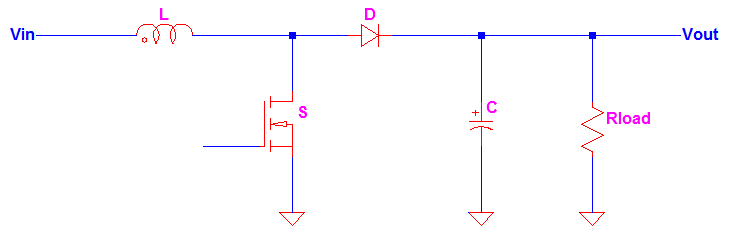## How to Select Inductor for Boost Converter

1. Select Inductance Value a. Define the switching frequency for the boost converter Example: Fsw = 300kHz b. Define the input and output voltage Example: Vin = 12V, Vout = 24V c. Determine the duty cycle Dutycycle = 1 – (Vin / Vout) Example: Dutycycle = 1 – (Vin / Vout) = 1 – (12V / 24V) = 50% d.## How to Calculate Boost Converter Ripple Current

In early stage of the design, boost converter ripple current is can be assumed as 25-40% of the RMS input current. But how to calculate boost converter ripple current from the design itself? This will be answered below, so better keep on reading. The 25-40% is just a pre-assumption value and can be changed depending on a specific need and